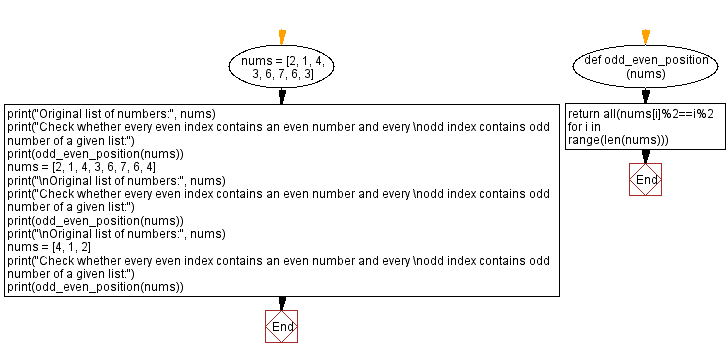﻿ Python: Check every even index contains an even number and odd index contains odd number of a given list - w3resource# Python: Check every even index contains an even number and odd index contains odd number of a given list

## Python Basic - 1: Exercise-95 with Solution

Write a Python program to check whether every even index contains an even number and every odd index contains odd number of a given list.

Sample Solution:

Python Code:

``````def odd_even_position(nums):
return all(nums[i]%2==i%2 for i in range(len(nums)))
nums = [2, 1, 4, 3, 6, 7, 6, 3]
print("Original list of numbers:", nums)
print("Check whether every even index contains an even number and every \nodd index contains odd number of a given list:")
print(odd_even_position(nums))
nums = [2, 1, 4, 3, 6, 7, 6, 4]
print("\nOriginal list of numbers:", nums)
print("Check whether every even index contains an even number and every \nodd index contains odd number of a given list:")
print(odd_even_position(nums))
print("\nOriginal list of numbers:", nums)
nums = [4, 1, 2]
print("Check whether every even index contains an even number and every \nodd index contains odd number of a given list:")
print(odd_even_position(nums))
``````

Sample Output:

```Original list of numbers: [2, 1, 4, 3, 6, 7, 6, 3]
Check whether every even index contains an even number and every
odd index contains odd number of a given list:
True

Original list of numbers: [2, 1, 4, 3, 6, 7, 6, 4]
Check whether every even index contains an even number and every
odd index contains odd number of a given list:
False

Original list of numbers: [2, 1, 4, 3, 6, 7, 6, 4]
Check whether every even index contains an even number and every
odd index contains odd number of a given list:
True
```

Pictorial Presentation:Flowchart:Python Code Editor:

Have another way to solve this solution? Contribute your code (and comments) through Disqus.

What is the difficulty level of this exercise?

Test your Programming skills with w3resource's quiz.

﻿

## Python: Tips of the Day

What is the difference between Python's list methods append and extend?

append: Appends object at the end.

```x = [1, 2, 3]
x.append([4, 5])
print (x)
```

Output:

```[1, 2, 3, [4, 5]]
```

extend: Extends list by appending elements from the iterable.

```x = [1, 2, 3]
x.extend([4, 5])
print (x)
```

Output:

```[1, 2, 3, 4, 5]
```

Ref: https://bit.ly/2AZ6ZFq